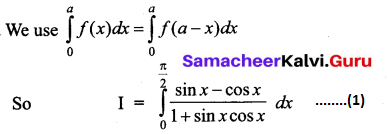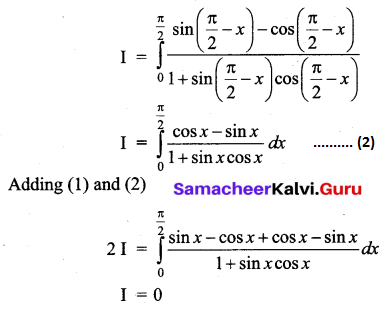I. One Mark Questions

Question 1.
The integral of $$\left(\sqrt{x}+\frac{1}{\sqrt{x}}\right)$$ equals _____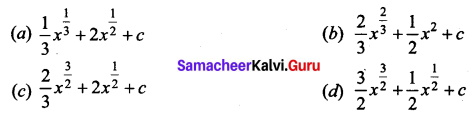(c) $$\frac{2}{3} x^{\frac{3}{2}}+2 x^{\frac{1}{2}}+c$$

Question 2.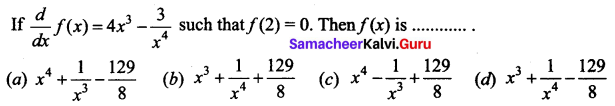(a) $$x^{4}+\frac{1}{x^{3}}-\frac{129}{8}$$
Hint: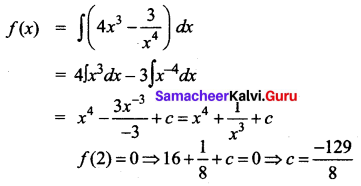Question 3.
$$\int \frac{\sin ^{2} x-\cos ^{2} x}{\sin ^{2} x \cos ^{2} x} d x$$ is equal to _____
(a) tan x + cot x + c
(b) tan x + cosec x + c
(c) -tan x + cot x + c
(d) tan x – sec x + c
(a) tan x + cot x + c
Hint: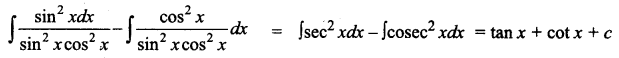Question 4.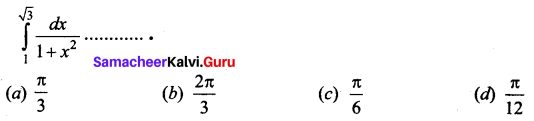(d) $$\frac{\pi}{12}$$
Hint: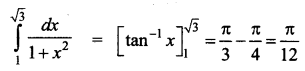Question 5.
The value of $$\int_{-\frac{\pi}{2}}^{\frac{\pi}{2}}\left(x^{3}+x \cos x+\tan ^{5} x\right) d x$$ is ______
(a) 0
(b) 2
(c) π
(d) 1
(a) 0
Hint:
Let f(x) = x3 + x cos x + tan5 x
f(-x)= -x3 – x cos x – tan5 x = -f(x)
So f(x) is odd function.
Integral is 0.

Question 6.
Fill in the blanks.
(a) $$\int_{0}^{\frac{\pi}{2}} \cos ^{3} x d x$$ is equal to _____
(b) $$\int_{-\frac{\pi}{2}}^{\frac{\pi}{2}} \sin ^{31} x d x$$ is equal to _________
(a) $$\frac{2}{3}$$
(b) 0
Hint: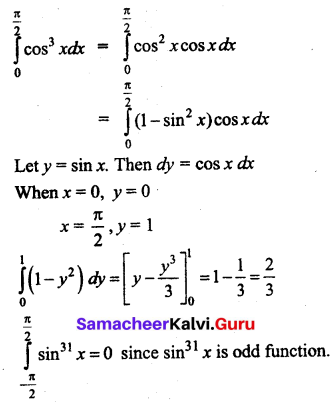Question 7.
Match the following.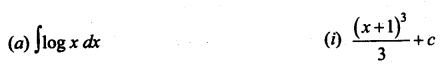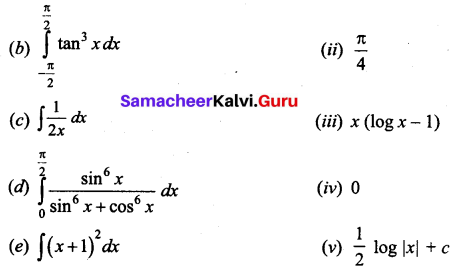(a) – (iii)
(b) – (iv)
(c) – (v)
(d) – (ii)
(e) – (i)

Question 8.
State True or False.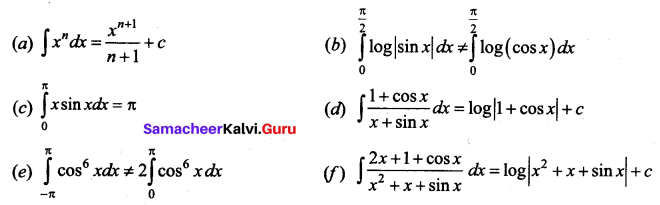(a) True
(b) False
(c) True
(d) False
(e) False
(f) True

Question 9.
Which of the following is not equal to ∫ tan x sec2 x dx?
(a) $$\frac{1}{2} \tan ^{2} x$$
(b) $$\frac{1}{2} \sec ^{2} x$$
(c) $$\frac{1}{2 \cos ^{2} x}$$
(d) None of these
(d) None of these
Hint: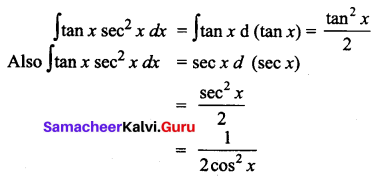Question 10.
∫ex (cos x – sin x) dx is equal to ______
(a) ex sin x + c
(b) ex cos x + c
(c) -ex cos x + c
(d) -ex sin x + c
(b) ex cos x + c
Hint:
Let f(x) = cos x
f'(x) = -sin x
∫ex [f(x) + f'(x)] dx = ex f(x) + c

Question 11.
$$\int \frac{1-\cos 2 x}{1+\cos 2 x} d x$$ is _____
(a) tan x – x + c
(b) x + tan x + c
(c) x – tan x + c
(d) -x – cot x + c
(a) tan x – x + c
Hint: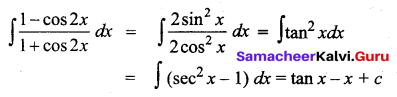Question 12.
$$\int_{0}^{\frac{\pi}{2}} \cos x e^{\sin x} d x$$ is equal to ______
(a) e = 1
(b) 1 – e
(c) $$e^{\frac{\pi}{2}}-1$$
(d) $$1-e^{\frac{\pi}{2}}$$
(a) e = 1
Hint: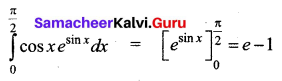Question 13.
Which of the following is an even function?
(a) sin x
(b) ex – e-x
(c) x cos x
(d) cos x
(d) cos x

Question 14.
Which of the following is neither odd nor even function?
(a) x sin x
(b) x2
(c) e-x
(d) x cos x
(c) e-x

Question 15.
∫sec2 (7 – 4x) dx equal to ______
(a) tan (7 – 4x)
(b) -tan (7 – 4x)
(c) –$$\frac{1}{4}$$ tan (7 – 4x)
(d) $$\frac{1}{4}$$ tan (7 – 4x)
(c) – $$\frac{1}{4}$$ tan (7 – 4x)

II. 2 Mark Questions.

Question 1.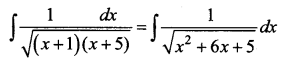Solution: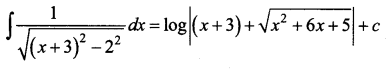Question 2.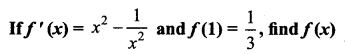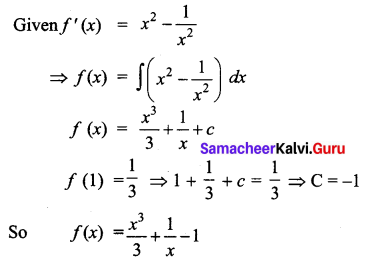Question 3.
$$\int\left(e^{x}+e^{-x}\right)^{2} d x$$
Solution: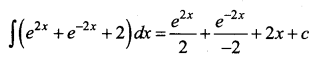Question 4.
Find $$\int x^{5} \sqrt{3+5 x^{6}} d x$$
Solution: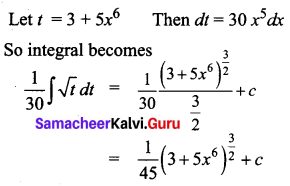Question 5.
$$\int \frac{(x+1)(x+\log x)^{2}}{x} d x$$
Solution: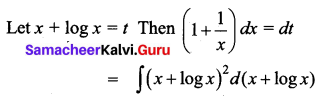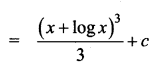Question 6.
$$\int \frac{e^{2 x}-1}{e^{2 x}+1} d x$$
Solution:
Dividing numerator and denominator by ex, we get $$\int \frac{e^{x}-e^{-x}}{e^{x}+e^{-x}} d x$$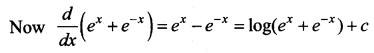Question 7.
$$\int \frac{1}{x+\sqrt{x}} d x$$
Solution: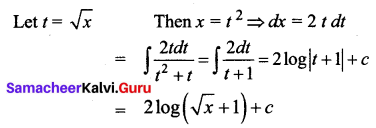III. 3 and 5 Mark Questions.

Question 1.
Find $$\int \frac{e^{x}(x+1)}{(x+3)^{3}} d x$$
Solution: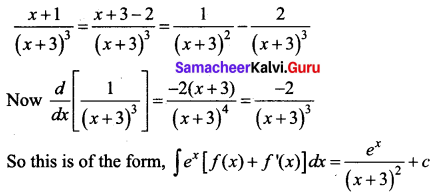Question 2.
$$\int \frac{1}{2 x^{2}-x-1} d x$$
Solution: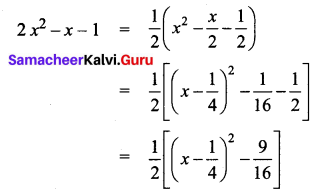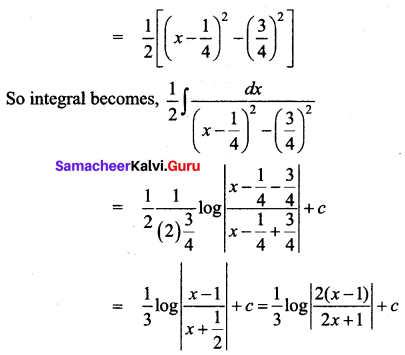Question 3.
$$\int \frac{1}{1-3 \sin ^{2} x} d x$$
Solution:
Divide the numerator and denominator by cos2 x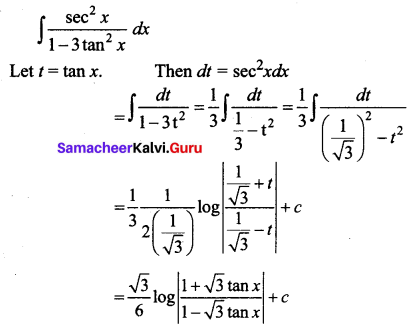Question 4.
$$\int \frac{d x}{e^{x}-e^{-x}}$$
Solution: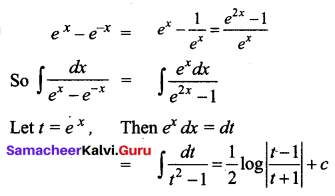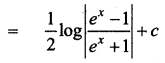Question 5.
Evaluate $$\int_{-1}^{2}(7 x-5) d x$$ as the limit of a sum.
Solution: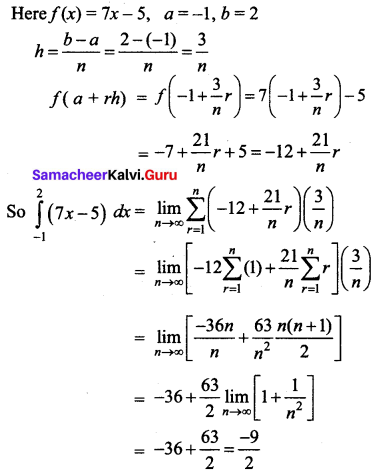Question 6.
Evaluate $$\int_{1}^{2}\left(x^{2}-1\right) d x$$ as the limit of a sum.
Solution: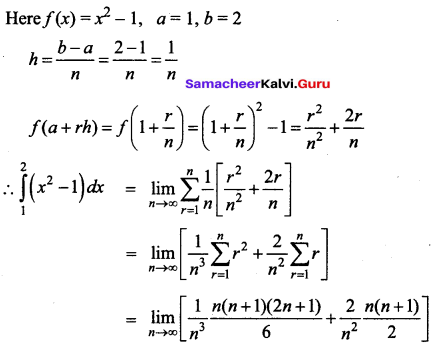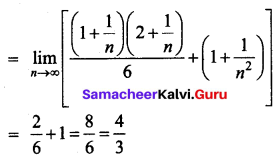Question 7.
Evaluate $$\int_{1}^{2} \frac{1}{x\left(x^{4}+1\right)} d x$$
Solution: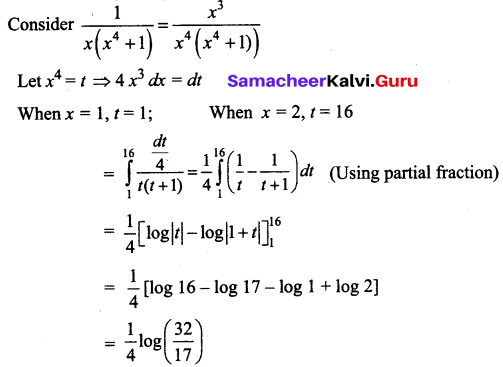Question 8.
Evaluate $$\int_{\frac{\pi}{6}}^{\frac{\pi}{3}} \frac{d x}{1+\sqrt{\tan x}}$$
Solution: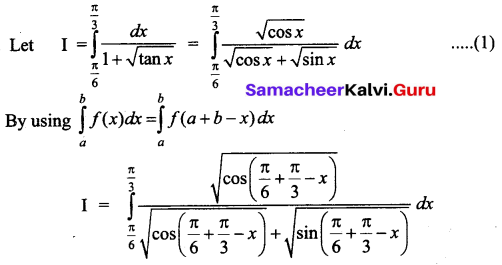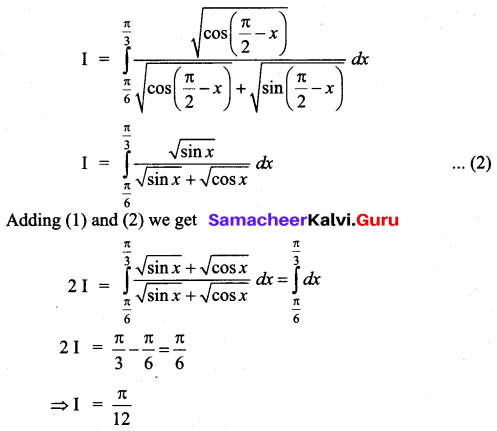Question 9.
Evaluate $$\int_{0}^{1} x(1-x)^{5} d x$$
Solution: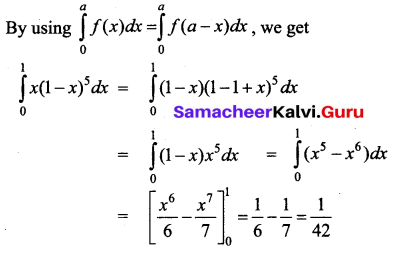Question 10.
Evaluate $$\int \frac{2 x+3}{\sqrt{x^{2}+x+1}} d x$$
Solution: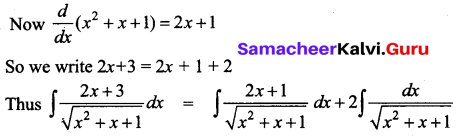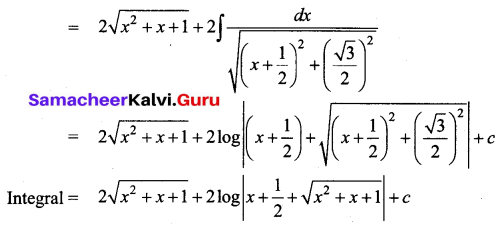Question 11.
∫(x2 + 1) log x dx
Solution: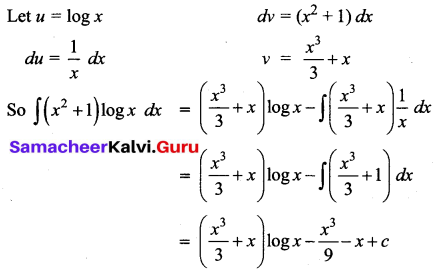Question 12.
$$\int \sqrt{x^{2}+4 x-5} d x$$
Solution: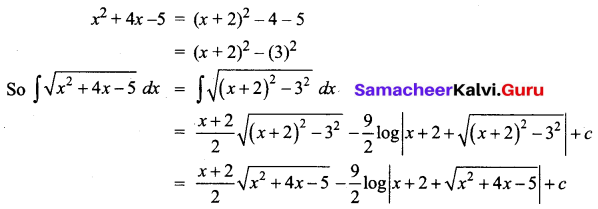Question 13.
$$\int_{0}^{\frac{\pi}{4}}\left(2 \sec ^{2} x+x^{3}+2\right) d x$$
Solution: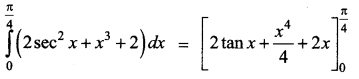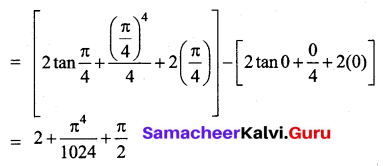Question 14.
Evaluate $$\int_{0}^{1} \frac{2 x}{\left(x^{2}+1\right)\left(x^{2}+2\right)} d x$$
Solution:
Let x2 = t, then 2x dx = dt
when x = 0, t = 0 and x = 1, t = 1
so integral becomes, $$\int_{0}^{1} \frac{d t}{(t+1)(t+2)}$$
We use partial fractions to proceed further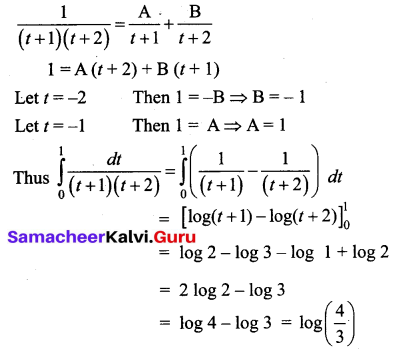Question 15.
$$\int_{0}^{\frac{\pi}{2}} \frac{\sin x-\cos x}{1+\sin x \cos x} d x$$
Solution: